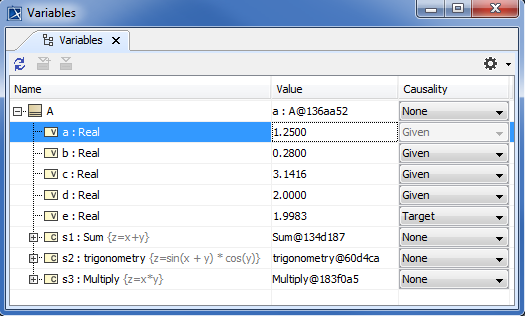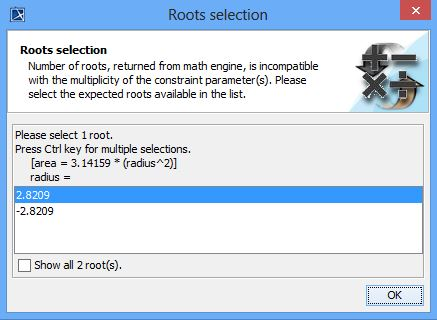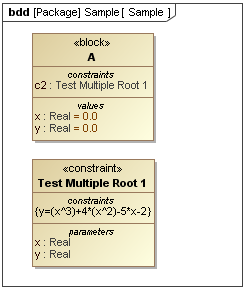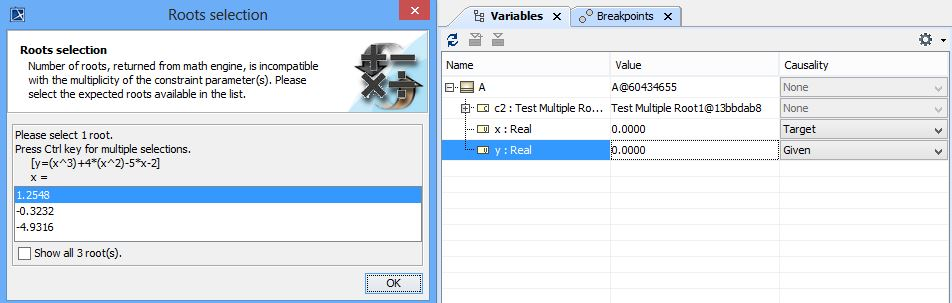Cameo Simulation Toolkit 19.0 LTR Documentation

As described in the section Mathematical equation, the parametric evaluator is capable of solving expressions in the mathematical equation to find the value of the target from the value of the given. We could say that the target is an unknown value that you want to find and the given is a known input value. Normally, a target is a variable on the left-hand side of an equation and a given is a variable on the right-hand side.

Sometimes, however, you know the value of the variable on the left-hand side of an equation and need to find the value of the variable on the right-hand side. You can use Cameo Simulation Toolkit to obtain the given variable if you integrate an external evaluator, which supports solving symbolic equations, to Cameo Simulation Toolkit. MATLAB with Symbolic Math Toolbox (see Integration with external Evaluators for more information about integrating with an external evaluator) is one of the external evaluators you can use.

If the language that defines an expression of a constraint block needs to be solved by an external evaluator that is capable of solving symbolic equations, you can specify what property is the target and the given through the Causality column in the Variables pane.

######Using MATLAB to evaluate mathematical equations.

The preceding figure shows the block object Circle in the Variables pane. It has a constraint property typed by the constraint block Circle Area. The expression of the constraint block is {area = 3.14159 *** (radius ^ 2)}. The default causality of area and radius will be target and given respectively. However, you can click the drop-down list box in the Causality column to change the causality of area and radius to be given and target. Cameo Simulation Toolkit will then evaluate the value of radius from the given value of area

The expression of the constraint block Circle Area in the figure shows two roots: (i) a positive value 2.8209 and (ii) a negative value -2.8209. The parametric evaluator needs only one root to evaluate the value of a radius from the given value of an area. Therefore, the Roots selection dialog will open for you to select which root you want as shown in the following figure.

######Roots Selection dialog.

The number of roots varies according to the expression and the multiplicity of a property, which is the target. Therefore, it is possible to select more than one root. The following scenarios show you how to work with multiple roots.

Scenario 1
The following figure shows the constraint Test Multiple Root 1 is applied to block A

######The Constraint Test Multiple Root 1 is Applied to Block A

Scenario 2
The given types of causality of y and x are given and target respectively. Once Cameo Simulation Toolkit finished executing block A and performing a parametric simulation to satisfy the constraint Test Multiple Root 1, the equation would result in three values of x and all are the possible roots for the equation. Therefore, the Roots selection dialog would open, allowing you to select one of these three values as the root (see the following figure).

######The Roots Selection Dialog.

Scenario 3
However, you may select more than one value as the root. But, the number of values you can select cannot be more than the upper multiplicity. Otherwise, an error will occur and an Error message dialog will open. The following figure shows that two values were selected as the roots when in fact, the upper multiplicity of x value property was one. Therefore, an error occurred and the Error dialog would open.

• No labels